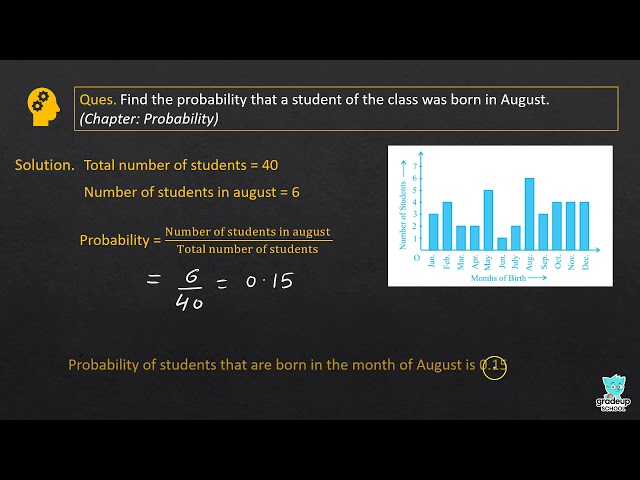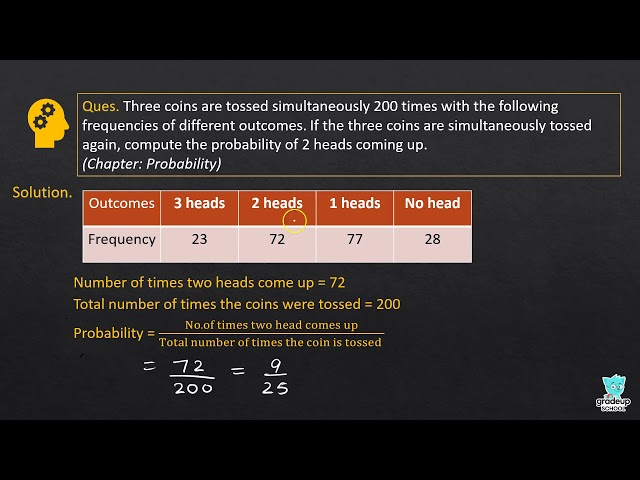# NCERT Solutions for Class 9 Maths Chapter 15 - Probability

Share

The NCERT Solutions For Class 9 Maths Chapter 15 – Probability that has been presented here is aimed at helping students learn the difficult concepts with ease. These Solutions for Chapter 14 have been prepared by expert teachers of Maths after conducting extensive research. Also, to make sure that these Solutions prove highly effective for students, they have strictly kept in sync with the present syllabus of the CBSE Class 9.

Chapter 15 – Probability of Maths textbook introduces to students the various concept of Probability and Probability – An Experimental Approach among others. Further, the Chapter discusses various problems on Probability. So, if you want to learn important topics and get easy answers to difficult questions, then our NCERT Solutions can help you a great deal. By referring to these Solutions for Chapter 15, you can practice important questions thoroughly and score good marks in your 9th Class CBSE exam.

## NCERT Solutions for Class 9 Maths Chapter 15 - Probability

Exercise 15.1
• Exercise 15.1
Class 9th|NCERT - MathematicsChapter 15 - ProbabilitySee More (3)
NCERT - MathematicsClass 9th , NCERT
 Chapter 1 - Number System Chapter 2 - Polynomials Chapter 3 - Coordinate Geometry Chapter 4 - Linear Equations in two Variables Chapter 5 - Introduction to Euclid's Geometry Chapter 6 - Lines and Angles Chapter 7 - Triangles Chapter 8 - Quadrilaterals Chapter 9 - Areas of Parallelograms and Triangles Chapter 10 - Circles Chapter 11 - Constructions Chapter 12 - Heron's Formula Chapter 13 - Surface Areas and Volumes Chapter 14 - Statistics Chapter 15 - Probability